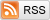# Logistic Regression Isn't Interpretable

March 13, 2017

Suppose two events A and B are independent, with the odds of A occurring being 4, and the odds of B being 5. What are the odds of both A and B occurring? I'll give you a hint: it's not 20.

Maybe you don't know or don't remember the definition of odds. Fair enough, let me explain or remind you: If an event occurs with probability p, then the odds of that event are defined to be p/(1-p), the ratio of the probability that the event occurs and the probability that it doesn't occur. With that out of the way, what are the odds that both A and B occur?

The correct answer is 2, ("two").

The way you figure this out is to first convert the odds above into probabilities. If the odds of A occurring are 4, then the probability that A occurs is 4/5. (0.8/(1-0.8) = 4). Similarly, the probability that B occurs is 5/6. The probability that both A and B occur is 4/5*5/6 = 2/3, the product of the probabilities that each occur, since A and B are independent. Finally, a probability of 2/3 corresponds to an odds of (2/3)/(1-2/3), or 2.

The point of this exercise is to demonstrate that odds are just not the way we typically think about randomness. If I mention "dice-rolling", I bet you immediately think "1/6" rather than "1/5" or "1:5". If I mention "presidential approval rating", I bet you think "42%" instead of "0.724" or even "42:58". People fundamentally think in terms of probabilities rather than odds.

There are at least two reasons why probabilities are easier to use than odds: Firstly, odds don't multiply, (as we saw above). The probability of that two independent events occur is the product of their marginal probabilities. More generally, P(A and B) = P(A|B) * P(B). The same isn't true for odds: multiplying two odds together has no easy interpretation. Secondly, odds don't add. If A and B are mutually exclusive events, then the probability that they both occur is the sum of their marginal probabilities. Again, there's no easy formula for odds.

The formulation of logistic regression in terms of log odds is the fundamental reason why logistic regression coefficients aren't interpretable. As a reminder, the logistic regression model specifies that you have n independent events, each of which occurs with some probability p, (a different p for each event). For some event with a vector of covariates x, the model specifies that log(p/(1-p)) = x^T b, where b is the vector of coefficients to be fit, typically with maximum likelihood. In other words, if you increase the value of some covariate by 1, the estimated log odds will increase by the corresponding coefficient.

So what does a coefficient of 0.3 mean, for example? The best concise explanation I can give is: "if you increase x by 1, the odds increase by 35%". (1.35 = exp(0.3)). But this is deeply unsatisfying: most people don't even know what odds are, (and would most likely misinterpret that explanation as being that the probability increases by 35 percentage points). And most of the people who do know what odds are likely don't have much of an intuition for what a 35% increase really means, since they tend to think in terms of probabilities.

Contrast this to linear regression, and the situation is completely different. Everyone knows what "each additional year of education was associated with an additional \$3000 in annual income" means. You can print that in a newspaper without any explanation. But try explaining a logistic regression coefficient to a lay audience without either confusing or misleading them.

Now, logistic regression isn't completely a black box: The linearity assumption means that you can compare the relative impact of the covariates by their coefficients, (assuming you've appropriately scaled the covariates). And the impact of changing a covariate depends only upon current estimated probability and the magnitude of the change, (in more complex models it can depend on the current values of all of the covariates). But I think there's still a lot of room for improvement. Given the popularity of binary outcome data, I think Statisticians should search for more interpretable models of binary outcomes.